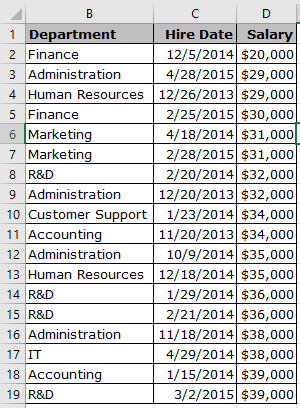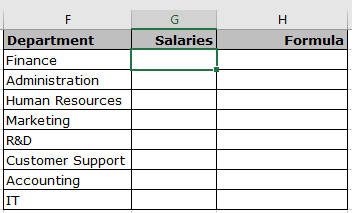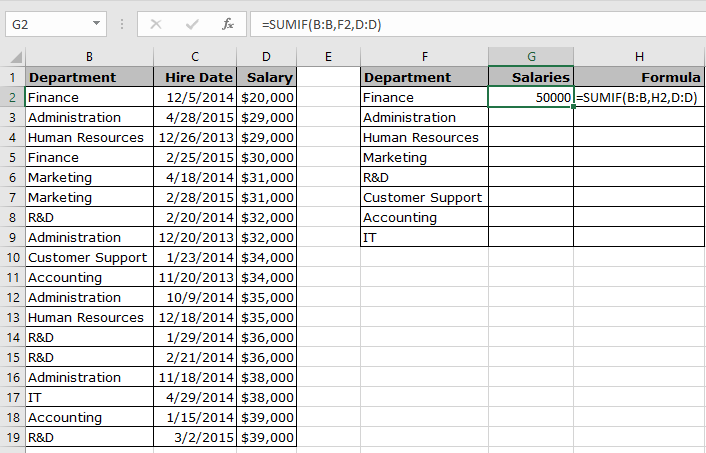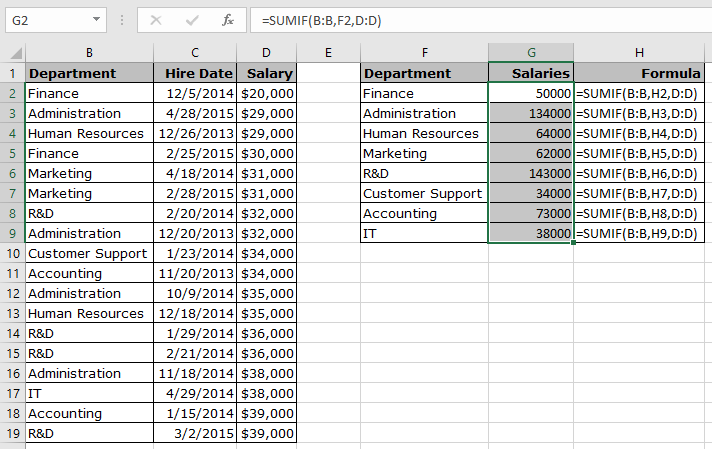# How to Sum if cells contain specific text in Excel

In this article, we will learn about how to Sum if cells contain specific text in Excel using SUMIF function.

In simple words, we need to find the sum corresponding to values if specific text matches

SUMIF function returns the sum of sum_range if text matches the text_range
Syntax:

=SUMIF(text_range, text, sum_range)

Let’s understand this function using it an example.Here we have a list of Department, Hire Date & Salaries.Now we will match the above department with the main table and find out the combined sum.

Use the formula

=SUMIF(B:B, F2, D:D)

Explanation:
String in F2 gets check with the Column B.
If the string matches with column B, corresponding values in Column D gets sum.As you can see in the above snapshot salaries corresponding to the “Finance” department gets sum.

Now we will use the formula for other departments.As you can see we got the sum if cells contain a specific text in Excel

Hope you understood how to get the sum if cells contain a specific text in Excel. Explore more articles on Excel functions here. Please feel free to state your query or feedback for the above article.

Related Articles:

How to Sum If Greater Than 0 in Excel

How to Sum if cell is not equal to value in Excel

How to Sum if date is greater than given date

How to Sum if cell contains text in other cell in Excel

Popular Articles

50 Excel Shortcut to Increase Your Productivity : Get faster at your task. These 50 shortcuts will make you work even faster on Excel.

How to use the VLOOKUP Function in Excel : This is one of the most used and popular functions of excel that is used to lookup value from different ranges and sheets.

How to use the COUNTIF function in Excel : Count values with conditions using this amazing function. You don't need to filter your data to count specific values. Countif function is essential to prepare your dashboard.

How to use the SUMIF Function in Excel : This is another dashboard essential function. This helps you sum up values on specific conditions.

1.